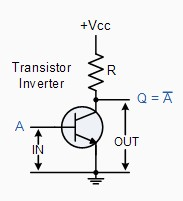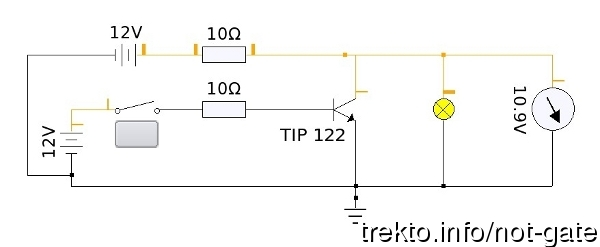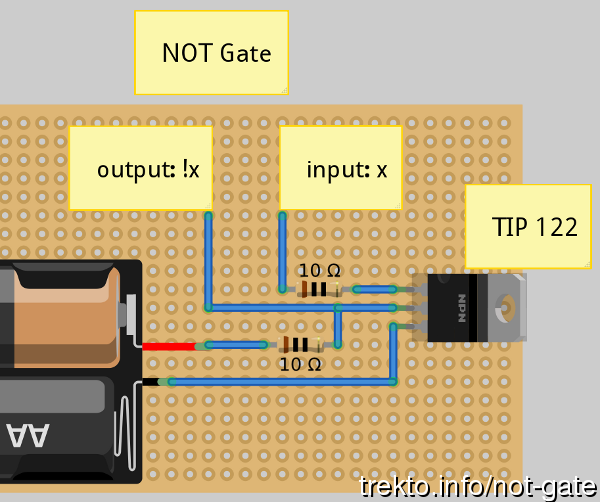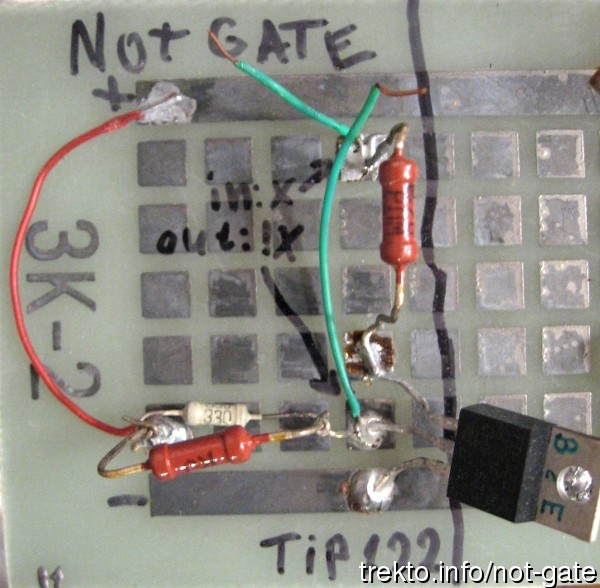# Simple "Not Gate" Scheme

23 Септ. 2012
Posted by trekto

Logic "Not Gate" or Digital Inverter is circuit which inverts the input.
If the input signal is true (1 or high) the output is false (0 or low). If the input is false (0 or low) the output is true (1 or high).
Not gate has a vast number of applications.

In my implementation I use TIP122 NPN transistor and 10Ω resistor but they can be replaced with other appropriate parts.

First I saw the Not Gate here: Logic "NOT" Gates where you can find a lot of information about different types of logic gates and specifically inverters. There I saw the following diagram:With KTechLab I made this circuit diagram:With Fritzing I made this scheme:And finally my implementation of the Not gate:Hope this is usefull.

## Публикувай нов коментар

• Адресите на уеб-страници и e-mail адресите автоматично се конвертират в хипервръзки.
• Разрешени HTML tag-ове: <a> <p> <span> <div> <h1> <h2> <h3> <h4> <h5> <h6> <img> <map> <area> <hr> <br> <br /> <ul> <ol> <li> <dl> <dt> <dd> <table> <tr> <td> <em> <b> <u> <i> <strong><font> <del> <ins> <sub> <sup> <quote> <blockquote> <pre> <address> <code> <cite> <embed> <object> <param> <strike> <caption>
• Линиите и параграфите се прекъсват автоматично.
• Mark language-dependent sections with == lc == where lc (or lc-xx) is a language code, other or all.

Повече информация за опциите на форматиране